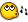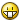# Get the repeated words !!

## Recommended Posts

Hi !!

First :: My English Is Bad , So I'm Sorry >_<

-----

I Want TO Get the repeated words !! In Array !!

Like !!

\$Array = [1, 2, 1, 2, 1]

I Want To Get

0+2+4

1+3

I tried this...

But It Need Some Thing```Local \$Array = [1, 2, 1, 2, 1]
;===============
Local \$x = 0, \$y = 0, \$RES
Do
Do
If \$Array[\$x] = \$Array[\$y] Then
\$RES &= \$x & "+" & \$y & "+"
EndIf
\$y += 1
Until \$y = UBound(\$Array)
\$RES = StringTrimRight(\$RES, 1) & "#"
\$x += 1
\$y = 0
Until \$x = UBound(\$Array)
\$RES = StringTrimRight(\$RES, 1)
;===============
MsgBox(0, "", \$RES)```

Thank you !!

##### Share on other sites

Hi !!

First :: My English Is Bad , So I'm Sorry-----

I Want TO Get the repeated words !! In Array !!

Like !!

\$Array = [1, 2, 1, 2, 1]

I Want To Get

0+2+4

1+3

I tried this...

But It Need Some Thing```Local \$Array = [1, 2, 1, 2, 1]
;===============
Local \$x = 0, \$y = 0, \$RES
Do
Do
If \$Array[\$x] = \$Array[\$y] Then
\$RES &= \$x & "+" & \$y & "+"
EndIf
\$y += 1
Until \$y = UBound(\$Array)
\$RES = StringTrimRight(\$RES, 1) & "#"
\$x += 1
\$y = 0
Until \$x = UBound(\$Array)
\$RES = StringTrimRight(\$RES, 1)
;===============
MsgBox(0, "", \$RES)```

Thank you !!

You should be able to change the output format of the \$ResDisp variable to whatever you want to display.

```;
Local \$Array = [6, "the", 1, 2, 1, 2, 6, 1, "the", 1, 6, 2]

Local \$ResDisp = "", \$ResTest = ""

For \$index = 0 To UBound(\$Array) - 2
If StringInStr(\$ResTest, \$Array[\$index]) = 0 Then
\$ResTest &= \$Array[\$index] & " "
\$ResDisp &= \$Array[\$index] & @TAB & " (" & \$index & "+"
\$y = \$index + 1
Do
If \$Array[\$index] = \$Array[\$y] Then
\$ResDisp &= \$y & "+"
EndIf
\$y += 1
Until \$y = UBound(\$Array)
\$ResDisp = StringTrimRight(\$ResDisp, 1) & ")" & @CRLF
EndIf
Next
\$ResDisp = StringTrimRight(\$ResDisp, 1)

MsgBox(0, "Results", \$ResDisp)
;```
##### Share on other sites

You should be able to change the output format of the \$ResDisp variable to whatever you want to display.

```;
Local \$Array = [6, "the", 1, 2, 1, 2, 6, 1, "the", 1, 6, 2]

Local \$ResDisp = "", \$ResTest = ""

For \$index = 0 To UBound(\$Array) - 2
If StringInStr(\$ResTest, \$Array[\$index]) = 0 Then
\$ResTest &= \$Array[\$index] & " "
\$ResDisp &= \$Array[\$index] & @TAB & " (" & \$index & "+"
\$y = \$index + 1
Do
If \$Array[\$index] = \$Array[\$y] Then
\$ResDisp &= \$y & "+"
EndIf
\$y += 1
Until \$y = UBound(\$Array)
\$ResDisp = StringTrimRight(\$ResDisp, 1) & ")" & @CRLF
EndIf
Next
\$ResDisp = StringTrimRight(\$ResDisp, 1)

MsgBox(0, "Results", \$ResDisp)
;```

WOW

Very Smart

Thank You !!

I am grateful to you ..

## Create an account

Register a new account

• ### Recently Browsing   0 members

×

• Wiki

• Back

• #### Beta

• Git
• FAQ
×
• Create New...